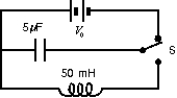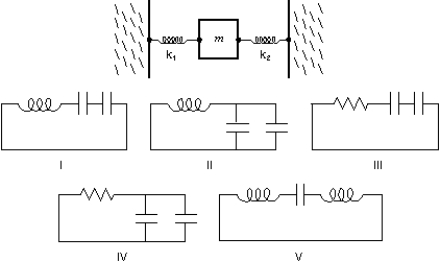# Fundamentals of Physics Study Set 2

Physics & Astronomy

## Quiz 31 : Electromagnetic Oscillations and Alternating CurrentStudy FlashcardsLooking for Introductory Physics Homework Help?

## Quiz 31 :Electromagnetic Oscillations and Alternating Current

Question TypeA charged capacitor and an inductor are connected in series.At time t = 0 the current is zero, but the capacitor is charged.If T is the period of the resulting oscillations, the next time, after t = 0 that the charge on the capacitor is a maximum is:
Free
Multiple Choice

B

Tags
Choose question tagAn LC series circuit with an inductance L and a capacitance C has an oscillation frequency f.If we now take two of those inductors, each with inductance L, and two of the capacitors, each with capacitance C, and wire them all in series to make a new circuit, its oscillation frequency will be:
Free
Multiple Choice

C

Tags
Choose question tagA capacitor in an LC oscillator has a maximum potential difference of 15 V and a maximum energy of 360 J.At a certain instant the energy in the capacitor is 40 J.At that instant what is the potential difference across the capacitor?
Free
Multiple Choice

B

Tags
Choose question tagAn LC circuit has an inductance of 15 mH and a capacitance of 10 F.At one instant the charge on the capacitor is 25 C.At that instant the current is changing at the rate:
Multiple Choice
Tags
Choose question tagThe electrical analog of a spring constant k is:
Multiple Choice
Tags
Choose question tagA charged capacitor and an inductor are connected in series.At time t = 0 the current is zero, but the capacitor is charged.If T is the period of the resulting oscillations, the next time, after t = 0 that the energy stored in the magnetic field of the inductor is a maximum is:
Multiple Choice
Tags
Choose question tagWe desire to make an LC circuit that oscillates at 100 Hz using an inductance of 2.5 H.We also need a capacitance of:
Multiple Choice
Tags
Choose question tagWhich of the following has the greatest effect in decreasing the oscillation frequency of an LC circuit? Using instead:
Multiple Choice
Tags
Choose question tagIn an oscillating LC circuit, the total stored energy is U and the maximum charge on the capacitor is Q.When the charge on the capacitor is Q/2, the energy stored in the inductor is:
Multiple Choice
Tags
Choose question tagA capacitor in an LC oscillator has a maximum potential difference of 15 V and a maximum energy of 360 J.At a certain instant the energy in the capacitor is 40 J.At that instant what is the emf induced in the inductor?
Multiple Choice
Tags
Choose question tagIn the circuit shown, switch S is first pushed up to charge the capacitor.When S is then pushed down, the current in the circuit will oscillate at a frequency of:Multiple Choice
Tags
Choose question tagA charged capacitor and an inductor are connected in series.At time t = 0 the current is zero, but the capacitor is charged.If T is the period of the resulting oscillations, the next time, after t = 0 that the energy stored in the electric field of the capacitor is a maximum is:
Multiple Choice
Tags
Choose question tagA charged capacitor and an inductor are connected in series.At time t = 0 the current is zero, but the capacitor is charged.If T is the period of the resulting oscillations, the next time, after t = 0 that the voltage across the inductor is a maximum is:
Multiple Choice
Tags
Choose question tagAn LC circuit has an oscillation frequency of 105 Hz.If C = 0.1 F, then L must be about:
Multiple Choice
Tags
Choose question tagAn LC circuit consists of a 1 F capacitor and a 4 mH inductor.Its oscillation frequency is approximately:
Multiple Choice
Tags
Choose question tagConsider the mechanical system consisting of two springs and a block, as shown.Which one of the five electrical circuits (I, II, III, IV, V)is the analog of the mechanical system?Multiple Choice
Tags
Choose question tagA 150-g block on the end of a spring with a spring constant of 35 N/m is pulled aside 25 cm and released from rest.In the electrical analog the maximum charge on the capacitor is 0.25 C.The maximum current in the LC circuit is:
Multiple Choice
Tags
Choose question tagAn LC circuit has an inductance of 20 mH and a capacitance of 5.0 F.If the charge amplitude is 40 C, what is the current amplitude?
Multiple Choice
Tags
Choose question tagA charged capacitor and an inductor are connected in series.At time t = 0 the current is zero, but the capacitor is charged.If T is the period of the resulting oscillations, the next time, after t = 0 that the current is a maximum is:
Multiple Choice